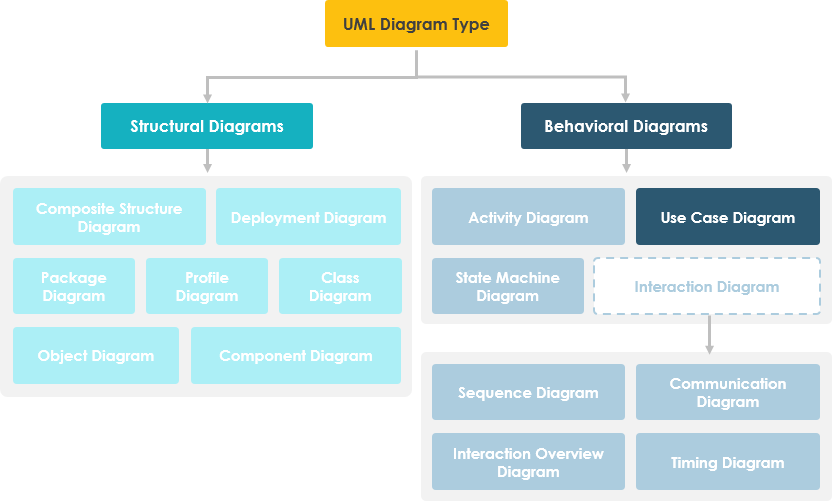1. Home
2. Docs
3. Chapter 5. UML & Requirement Diagram
4. 1. Use Case Diagram

# 1. Use Case Diagram

use case diagram at its simplest is a representation of a user’s interaction with the system that shows the relationship between the user and the different use cases in which the user is involved. A UML use case diagram is the primary form of system/software requirements for a new software program under developed. Use cases specify the expected behaviour (what), and not the exact method of making it happen (how). Use cases once specified can be denoted both textual and visual representation (such as UML). A key concept of use case modeling is that it helps us design a system from end user’s perspective. It is an effective technique for communicating system behavior in the user’s terms by specifying all externally visible system behavior. Thus, a use case diagram is the blueprints for your system, it provides the simplified and graphical representation of what the system must actually do.

While a use case itself might drill into a lot of detail about every possibility, a use-case diagram can help provide a higher-level view of the system.  it only summarizes some of the relationships between use cases, actors, and systems. In particular, the diagram does not show the order in which steps are performed to achieve the goals of each use case. You can describe those details in other diagrams and documents, which you can link to each use case.

A use case diagram is usually simple. It does not show the detail of the use cases:

• It only summarizes some of the relationships between use cases, actors, and systems.
• It does not show the order in which steps are performed to achieve the goals of each use case.

As said, a use case diagram should be simple and contains only a few shapes. If yours contain more than 20 use cases, you are probably mis-using usecase diagram.

The figure below shows the UML diagram hierarchy and the positioning of UML Use Case Diagram. As you can see, use case diagrams belong to the family of behavioural diagrams.